# Series-parallel R, L, and C with a 50 Ohm Signal Generator

#### chiques

Joined Feb 18, 2011
9
How does the math in the example RLC Example change when the 12V/60Hz source has a 50 Ohm impedance?

#### RoofSheep

Joined Mar 7, 2023
36
Just place a 50 Ohm resistor in series with the voltage source and repeat the analysis.

#### MrAl

Joined Jun 17, 2014
10,590
How does the math in the example RLC Example change when the 12V/60Hz source has a 50 Ohm impedance?
Hi,

Probably the first thing i would do is combine the 50 Ohm resistor with the 4.7uf capacitor:
Z=50+1/(j*w*4.7e-6)

#### chiques

Joined Feb 18, 2011
9

#### ericgibbs

Joined Jan 29, 2010
17,981
It looks like there be a larger voltage drop across the 50 Ohm + C1 in serial.
Hi c,
What numerical value do you calculate for the R and C in Series.?
E

#### MrAl

Joined Jun 17, 2014
10,590

#### chiques

Joined Feb 18, 2011
9

#### chiques

Joined Feb 18, 2011
9
hello again,

What are you trying to solve for? Is it the voltage across the 470 Ohm resistor?
Hi,
I'm trying to find all the voltages and current across each element using a 50 Ohm signal generator.

#### MrAl

Joined Jun 17, 2014
10,590

#### chiques

Joined Feb 18, 2011
9
Hi,

I checked your series calculation for the 50 Ohm and 4.7uf and it looks correct.
To check your other calculations and others like this you could use a circuit simulator. You should get results that match pretty close to the simulator results for problems like these.
The 50 Ohm resistor from the source doesn't seem to affect the circuit too much: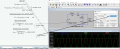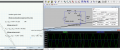I think I can live with a 5-10% error. It looks as if I can just neglect the 50 Ohm source impedance and move on with my calculations. But then again, 10% of 1000V is 100V :/

Last edited:

#### MrAl

Joined Jun 17, 2014
10,590
The 50 Ohm resistor from the source doesn't seem to affect the circuit too much:
View attachment 294759

View attachment 294760

I think I can live with a 5-10% error. It looks as if I can just neglect the 50 Ohm source impedance and move on with my calculations. But then again, 10% of 1000V is 100V :/
Hi,

Yes, it appears that it only makes a little difference, and the way a lot of people like to do it is to take the square of the real part and add it to the square of the imaginary part, and then take the square root of that result. When we do that here with 50 and 564, we get 566, which is just a little higher than what it was before (564 with no 50 Ohm series resistor).
So we go from roughly:
I=V/564
to:
I=V/566
where V is the voltage across either the 4.7uf cap, or the 4.7uf cap in series with the 50 Ohm resistor after the modification.
Note when we square the numbers the 564 becomes much more significant than the 50, because the larger number grows much more than the smaller.
The ratio 50/564=0.089 (close to 9 percent),
the ratio 50^2/564^2=0.0079 (close to 0.8 percent, a 10 fold decrease.
So it's not even 10 percent, looks much less than that, much less, 1 percent.
Seems ok to me.

The best test is to calculate the impedance of the circuit to the right of the 4.7uf cap, then compare that to the 4.7uf impedance, then the series 50 Ohm + 4.7uf impedance. Another test is to calculate the voltage across the Load both ways also, then compare. The voltage should not drop much.

Last edited:
•chiques

#### chiques

Joined Feb 18, 2011
9
Hi,

Yes, it appears that it only makes a little difference, and the way a lot of people like to do it is to take the square of the real part and add it to the square of the imaginary part, and then take the square root of that result. When we do that here with 50 and 564, we get 566, which is just a little higher than what it was before (564 with no 50 Ohm series resistor).
So we go from roughly:
I=V/564
to:
I=V/566
where V is the voltage across either the 4.7uf cap, or the 4.7uf cap in series with the 50 Ohm resistor after the modification.
Note when we square the numbers the 564 becomes much more significant than the 50, because the larger number grows much more than the smaller.
The ratio 50/564=0.089 (close to 9 percent),
the ratio 50^2/564^2=0.0079 (close to 0.8 percent, a 10 fold decrease.
So it's not even 10 percent, looks much less than that, much less, 1 percent.
Seems ok to me.

The best test is to calculate the impedance of the circuit to the right of the 4.7uf cap, then compare that to the 4.7uf impedance, then the series 50 Ohm + 4.7uf impedance. Another test is to calculate the voltage across the Load both ways also, then compare. The voltage should not drop much.
I separated them but the impedances are way off: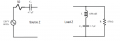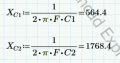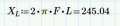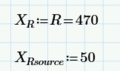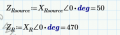Rsource ≠Rload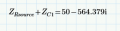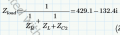#### MrAl

Joined Jun 17, 2014
10,590
I separated them but the impedances are way off:
View attachment 294811

View attachment 294812

View attachment 294813

View attachment 294814

View attachment 294815

View attachment 294816

Rsource ≠RloadView attachment 294817

View attachment 294818

Hello again,

I meant compare impedances on the left side to the right side with the 4.7uf, then
compare the left side to the right side with the 50 Ohm plus 4.7uf.
However, computing the voltage across R is even better.
With no 50 Ohm the output is 0.55 times the input voltage, and when we add the 50 Ohm the output is 0.53 times the input voltage.
That ratio is leaves us with a drop in voltage across R of about 4 percent when we add the 50 Ohm resistor.
Not too bad i think, but you have to decide that based on what exactly the application has to do.

You should try to verify this.